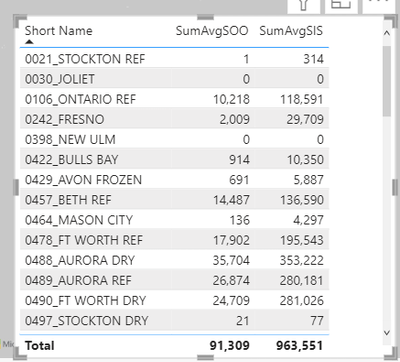cancel
Showing results for
Did you mean:Regular Visitor

## Would like a sum total for an average column

Hi all,

I have a "stupid" problem : I have to sum an average cost column.

Example :

I have actuallyAll values are averages but, for my need, the total row is wrong. I don't want 2 for 2020 and 3 for 2021 but 6 for 2020 and 9 for 2021.

That means :It will be validated too without rows headers.1 ACCEPTED SOLUTIONSuper User

Iterate the average calculate at the desired granularity and add it all up.

``````SUMX (
VALUES ( Table1[ColABC] ),
CALCULATE ( AVERAGE ( Table1[Value] ) )
)``````

Or if you already have an averaging measure:

``SUMX ( VALUES ( Table1[ColABC] ), [AvgMeasure] )``

4 REPLIES 4Super User

Iterate the average calculate at the desired granularity and add it all up.

``````SUMX (
VALUES ( Table1[ColABC] ),
CALCULATE ( AVERAGE ( Table1[Value] ) )
)``````

Or if you already have an averaging measure:

``SUMX ( VALUES ( Table1[ColABC] ), [AvgMeasure] )``New Member

I tried that equation but my grand total is wrong.

average_soo = AVERAGE(CONTROL_TOWER_TIER1_TIER2_SUMMARY_FACT[STORE_OOS])
average_ssi = AVERAGE(CONTROL_TOWER_TIER1_TIER2_SUMMARY_FACT[STORE_IN_STOCK])

SumAvgSIS = SUMX(VALUES(MATERIAL_DIM[MATERIAL]),[average_ssi])
SumAvgSOO = SUMX(VALUES(MATERIAL_DIM[MATERIAL]),[average_soo])New Member

Searched a lot for this answer, thanks for it!Regular Visitor

Hi Alexis,

Thanks a lot. It works fine

😀